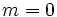# Ree group:Ree(3)

Jump to: navigation, search
This article is about a particular group, i.e., a group unique upto isomorphism. View specific information (such as linear representation theory, subgroup structure) about this group
View a complete list of particular groups (this is a very huge list!)[SHOW MORE]

## Definition

This group can be defined in two equivale ways:

1. It is the Ree group with parameter value 3, i.e., the group$\operatorname{Ree}(3)$. Equivalently, it is the Ree group$Ree(3^{1+2m})$ where$m = 0$. It is the only Ree group that is not simple. The Ree groups$\operatorname{Ree}(3^{1 + 2m})$,$m > 0$, are all simple.
2. It is the projective semilinear group of degree two over field:F8, i.e., it is the group$P\Gamma L(2,8)$.

## Arithmetic functions

Function Value Similar groups Explanation
order (number of elements, equivalently, cardinality or size of underlying set) 1512 groups with same order As$\operatorname{Ree}(q)$,$q = 3$:$q^3(q^3 + 1)(q - 1) = 3^3(3^3 + 1)(3 - 1) = 27(28)(2) = 1512$
As$P\Gamma L(2,q), q = 8 = 2^3, p = 2, r = 3$:$r(q^3 - q) = 3(8^3 - 8) = 3(504) = 1512$
exponent of a group 126 groups with same order and exponent of a group | groups with same exponent of a group
number of conjugacy classes 11 groups with same order and number of conjugacy classes | groups with same number of conjugacy classes

### Arithmetic functions of a counting nature

Function Value Explanation
number of conjugacy classes 11
number of conjugacy classes of subgroups 25
number of subgroups 1154

## GAP implementation

### Group ID

This finite group has order 1512 and has ID 779 among the groups of order 1512 in GAP's SmallGroup library. For context, there are 889 groups of order 1512. It can thus be defined using GAP's SmallGroup function as:

SmallGroup(1512,779)

For instance, we can use the following assignment in GAP to create the group and name it$G$:

gap> G := SmallGroup(1512,779);

Conversely, to check whether a given group$G$ is in fact the group we want, we can use GAP's IdGroup function:

IdGroup(G) = [1512,779]

or just do:

IdGroup(G)

to have GAP output the group ID, that we can then compare to what we want.

### Other descriptions

Description Functions used
Ree(3) Ree